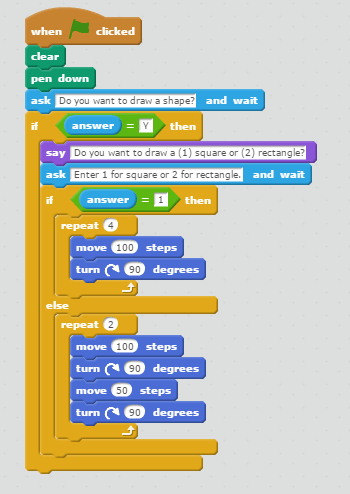# Making Decisions

10.07.2017

Episode #4 of the course Coding сoncepts with Scratch by Alan Cohen

Making decisions is an important part of programming, as it lets a program do different things based on what the user enters. For example, an online survey asks if you are married or single. Your answer determines which set of questions display.

This lesson introduces you to how programs make decisions. You will learn how to use the If-Then block and the If-Then-Else block.

If-Then Block

The If-Then block performs a block or set of blocks if the condition is true. If the condition is false, the code ignores these block(s). The program continues with the blocks that follow the If-Then block. Let’s look at an example.

The following code asks the user if they want to draw a square. If the user answers “Y” for yes, the program draws a square and then displays the message, “Have a nice day!” If the user answers “N” for no, the program ignores the blocks that draw a square and displays the message, “Have a nice day!”If-Then-Else block

The If-Then-Else block performs one set of blocks if the condition is true and another if the condition is false. The following example expands on our previous example. The program asks the user if they want to draw a square. If the user responds “Y” for yes, the program draws a square and displays the message, “Here is your square.” If the user responds “N” for no, the program draws a rectangle and displays the message, “I drew a rectangle for you.” In both cases, the program displays the message, “Have a nice day!”

The first part of the program is almost the same as our If-Then example. The only difference is that the program displays the message, “Here is your square.” It then leaves the If-Then-Else block and displays the message, “Have a nice day!”

The Else section executes if the user entered “N” for no. The code in this section draws a rectangle and displays the message, “I drew a rectangle for you.” It then leaves the If-Then-Else block and displays the message, “Have a nice day!”You can code more decision-making logic by including If-Then and If-Then-Else blocks within each other. The following program also asks the user to draw either a square or a rectangle. This example includes an If-Then-Else block within an If-Then block. Programs can include as many If-Then and If-Then-Else blocks as needed.

Our example begins by asking the user if they want to draw a shape. If the user enters “N” for no, the program ends. If the user enters “Y” for yes, the program asks the user if they want to draw a square or a rectangle. The user enters “1” to draw a square and “2” to draw a rectangle.Congratulations! You learned how programs make decisions! The next lesson shows you how to use variables to store and work with information.

Recommended book

DK Workbooks: Coding with Scratch Workbook by DK

Share with friends